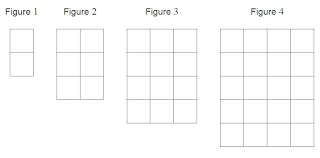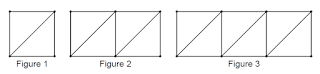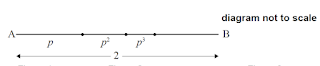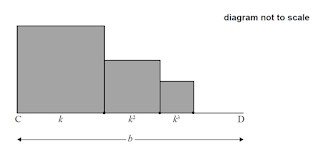# AP and GP (IB SL)

$\def\D{\displaystyle}\def\frac{\dfrac}$
 $\quad$ 1 (IB/sl/2019/November/Paper1/q1) [Maximum mark: 6] In an arithmetic sequence, $u_{2}=5$ and $u_{3}=11$. (a) Find the common difference. (b) Find the first term.  (c) Find the sum of the first 20 terms. 2 (IB/sl/2018/November/Paper1/43) [Maximum mark: 6] In an arithmetic sequence, $u_{1}=-5$ and $d=3$. (a) Find $u_{\mathrm{g}}$. (b) Find the value of $n$ for which $u_{n}=67$. 3 (IB/sl/2018/May/paper2tz1/q7) [Maximum mark: 8] Let $f(x)=e^{\sin \left(\frac{\pi}{2}\right)}$, for $x>0.$ The $k$ th maximum point on the graph of $f$ has $x$-coordinate $x_{k}$ where $k \in \mathbb{Z}^{+} .$ (a) Given that $x_{k+1}=x_{k}+a$, find $a$. (b) Hence find the value of $n$ such that $\D\sum_{k=1}^{v} x_{k}=861$. 4 (IB/sl/2017/November/Paper1/42) [Maximum mark: 6] In an arithmetic sequence, the first term is 8 and the second torm is $5 .$ (a) Find the common difference. (b) Find the tenth term.  (c) Find the sum of the first ten terms.  5 (IB/sl/2017/May/paper1tz2/q1) [Maximum mark: 6] In an arithmetic sequence, the first term is 3 and the second term is 7 . (a) Find the common difference.  (b) Find the tenth term.  (c) Find the sum of the first ten terms of the sequence.  6 (IB/sl/2016/May/paper2tz2/q1) [Maximum mark: 6] The first three terms of an arithmetic sequence are $u_{1}=0.3, u_{2}=1.5, u_{3}=2.7$, (a) Find the common difference. (b) Find the 30 th term of the sequence. $$ (c) Find the sum of the first 30 terms. 7 (IB/sl/2017/May/paper1tz2/q4) [Maximum mark: 6] The following figures consist of rows and columns of squares. The figures form a continuing pattern. Figure 1 has two rows and one column. Figure 2 has three rows and two columns.Figure 5 , has $p$ rows and $q$ columns. (a) Write down the value of (i) $p$; (ii) $q$,  Each small square has an area of $1 \mathrm{~cm}^{2}$. Let $A_{v}$ be the total area of Figure $n$. The following table gives the first five values of $A_{\mathrm{n}}$. $$\begin{array}{|c|c|c|c|c|c|}\hline n & 1 & 2 & 3 & 4 & 5 \\\hline A_{n}\left(\mathrm{~cm}^{2}\right) & 2 & 6 & 12 & 20 & k \\\hline\end{array}$$ (b) Find the value of $k$.  (c) Find an expression for $A_{p}$ in terms of $n$.  8 (IB/sl/2016/May/paper1tz1/q4) [Maximum mark: 6] Consider the following sequence of figures.Figure 1 contains 5 line segments. (a) Given that Figure $n$ contains 801 line segments, show that $n=200$. (b) Find the total number of line segments in the first 200 figures.  9 (IB/s1/2019/November/Paper2/q5) [Maximum mark: 7] The first two terms of a geometric sequence are $u_{1}=2.1$ and $u_{2}=2.226$. (a) Find the value of $r$. (b) Find the value of $u_{10}$. (c) Find the least value of $n$ such that $S_{n}>5543$.  10 (IB/s1/2019/May/paper2tz1/q7) [Maximum mark: 7] The first terms of an infinite geometric sequence, $u_{v}$, are $2,6,18,54, \ldots .$ The first terms of a second infinite geometric sequence, $v_{n}$, are $2,-6,18,-54, \ldots .$ The terms of a third sequence, $w_{n}$, are defined as $w_{n}=u_{n}+v_{n}$ (a) Write down the first three non-zero terms of $w_{n}$. The finite series, $\D\sum_{k=1}^{23 x} w_{k}$, can also be written in the form $\D\sum_{k=0}^{n} 4 r^{k}$. (b) Find the value of (i) $r i$ (ii) $m$, $$ 11 [Maximum mark: 16] In an arithmetic sequence, $u_{1}=1.3, u_{2}=1.4$ and $u_{k}=31.2$. (a) Find the value of $k$. (b) Find the exact value of $S_{R}$.  Consider the terms, $u_{x}$, of this sequence such that $n \leq k$. Let $F$ be the sum of the terms for which $n$ is not a multiple of 3 . (c) Show that $F=3240$. An infinite geometric series is given as $S_{\infty}=a+\frac{a}{\sqrt{2}}+\frac{a}{2}+\ldots, a \in \mathbb{Z}^{+}$. (d) Find the largest value of $a$ such that $S_{n} < F$. 12 (IB/sl/2018/November/Paper2/q5) [Maximum mark: 6] The sum of an infinite geometric sequence is $33.25$. The second term of the sequence is $7.98$. Find the possible values of $r$. 13 (IB/sl/2018/May/paper1tz1/q10) [Maximum mark: 15] The first two terms of an infinite geometric sequence are $u_{1}=18$ and $u_{2}=12 \sin ^{2} \theta$. where $0 < \theta < 2 \pi$, and $\theta$. (a) (i) Find an expression for $r$ in terms of $\theta$. (ii) Find the possible values of $r$. (b) Show that the sum of the infinite sequence is $\frac{54}{2+\cos (2 \theta)}$. (c) Find the values of $\theta$ which give the greatest value of the sum. 14 (IB/sl/2018/May/paper2tz2/q4) [Maximum mark: 7] The first term of an infinite geometric sequence is 4 . The sum of the infinite sequence is 200 . (a) Find the common ratio. (b) Find the sum of the first 8 terms.  (c) Find the least value of $n$ for which $S_{v}>163$.  15 (IB/s1/2017/November/Paper1/q10) [Maximum mark: 14] (a) The following diagram shows [AB], with length $2 \mathrm{~cm}$. The line is divided into an infinite number of line segments. The diagram shows the first three segments.The length of the line segments are $p \mathrm{~cm}, p^{2} \mathrm{~cm}, p^{3} \mathrm{~cm}, \ldots .$ where $0 < p < 1$ Show that $p=\frac{2}{3}$. (b) The following diagram shows [CD], with length $b \mathrm{~cm}$, where $b>1$. Squares with side, lengths $k \mathrm{~cm}, k^{2} \mathrm{~cm}, k^{3} \mathrm{~cm}, \ldots$, where $0 < k < 1$, are drawn along [CD]. This process is carried on indefinitely. The diagram shows the first three squares.The total sum of the areas of all the squares is $\frac{9}{16}$. Find the value of $b$. 16 (IB/sl/2017/May/paper1tz1/q7) [Maximum mark: 8] The first three terms of a geometric sequence are $\ln x^{16}, \ln x^{8}, \ln x^{4}$, for $x>0$, (a) Find the common ratio. (b) Solve $\D\sum_{k=1}^{\infty} 2^{5-x} \ln x=64$. 17 (IB/sl/2017/May/paper2tz2/q5) [Maximum mark: 6] Consider a geometric sequence where the first term is 768 and the second term is $576 .$ Find the least value of $n$ such that the $n$th term of the sequence is less than 7 . 18 (IB/sl/2016/November/Paper1/q9) [Maximum mark: 13] The first two terms of an infinite geometric sequence, in order, are $2 \log _{2} x, \log _{2} x$, where $x>0$ (a) Find $r$. (b) Show that the sum of the infinite sequence is $4 \log _{2} x$. The first three terms of an arithmetic sequence, in order, are $$\log _{2} x, \log _{2}\left(\frac{x}{2}\right), \log _{2}\left(\frac{x}{4}\right), \text { where } x>0$$ (c) Find $d$, giving your answer as an integer. Let $S_{12}$ be the sum of the first 12 terms of the arithmetic sequence, (d) Show that $S_{12}=12 \log _{2} x-66$. (e) Given that $S_{12}$ is equal to half the sum of the infinite geometric sequence, find $x$, giving your answer in the form $2^{p}$, where $p \in \mathbb{Q}$. 19 (IB/sl/2016/May/paper1tz2/q4) [Maximum mark: 6] Three consecutive terms of a geometric sequence are $x-3,6$ and $x+2$. Find the possible values of $x$. 20 (IB/sl/2016/May/paper2tz1/q6) [Maximum mark: 6] In a geometric sequence, the fourth term is 8 times the first term. The sum of the first 10 terms is $2557.5$. Find the 10 th term of this sequence.

21 (IB/sl/2015/May/paper2tz1/q3)
[Maximum mark: 6]
In an arithmetic sequence $u_{10}=8, u_{11}=6.5$.
 (a) Write down the value of the common difference.
 (b) Find the first term.
 (c) Find the sum of the first 50 terms of the sequence.

 22 (IB/sl/2015/November/Paper1/q7) [Maximum mark: 6] An arithmetic sequence has the first term $\ln a$ and a common difference $\ln 3$. The 13 th term in the sequence is $8 \ln 9 .$ Find the value of $a .$

 23 (IB/sl/2015/May/paper2tz2/q6) [Maximum mark: 7 ] Ramiro walks to work each morning. During the first minute he walks 80 metres. In each subsequent minute he walks $90 \%$ of the distance walked during the previous minute. The distance between his house and work is 660 metres. Ramiro leaves his house at $08: 00$ and has to be at work by $08: 15 .$ Explain why he will not be at work on time.

24 (IB/sl/2015/November/Paper2/g4)
[Maximum mark: 7]
The first three terms of a geometric sequence are $u_{1}=0.64, u_{2}=1.6$, and $u_{3}=4 .$
 (a) Find the value of $r$.
 (b) Find the value of $S_{6}$.
 (c) Find the least value of $n$ such that $S_{n}>75000$. 

1 (a) 6 (b) $-1$ (c) 1120

2 (a) $u_{8}=16$ (b) $n=25$

3 (a) $a=4$ (b) $\quad n=21$

4 (a) $d=-3$ (b) $u_{10}=-19$ (c) $S_{10}=-55$

5 (a) $d=4$ (b) $u_{10}=39$ (c) $S_{10}=210$

6 (a) $d=1,2$ (b) $u_{30}=35.1$ (c) $S_{30}=531$

7 (a) (i) $p=6$ (ii) $q=5$ (b) $k=30$ (c) $A_{n}=n(n+1)$

8 (a) $n=200$ (b) 80600

9 (a) 1.06 (b) 3.55 (c) 88

10 (a) 4,36,324 (b)(i) 9 (ii) 112

11 (a) $k=300$ (b) $S_{k}=4875$ (c) Show (d) 948

12 $\quad r=\frac{2}{5}, r=\frac{3}{5}$

13 (a)(i) $\quad r=\frac{2 \sin ^{2} \theta}{3}$ (ii) $0<r \leqslant \frac{2}{3}$ (b) Show (c) $\theta=\frac{\pi}{2}, \frac{3 \pi}{2}$

14 (a) $\quad r=0.98$ (b) $29.8$ (c) $n=84$

15 (a) Show (b) $b=\frac{3}{2}$

16 (a) $r=\frac{1}{2}$ (b) $x=e^{2}$

17 $n=18$

18 (a) $r=\frac{1}{2}$ (b) Show (c) $d=-1$ (d) Show (e) $x=2^{6 .6}$

19 $x=7, x=-6$

20 1280

21(a) $d=-1.5$ (b) $u_{1}=21.5$ (c) $-762.5$
22 $\quad a=81$
23 $n=16.5>15$, Late
24(a) $r=2.5$ (b) $S_{6}=103.74$ (c) $n=14$